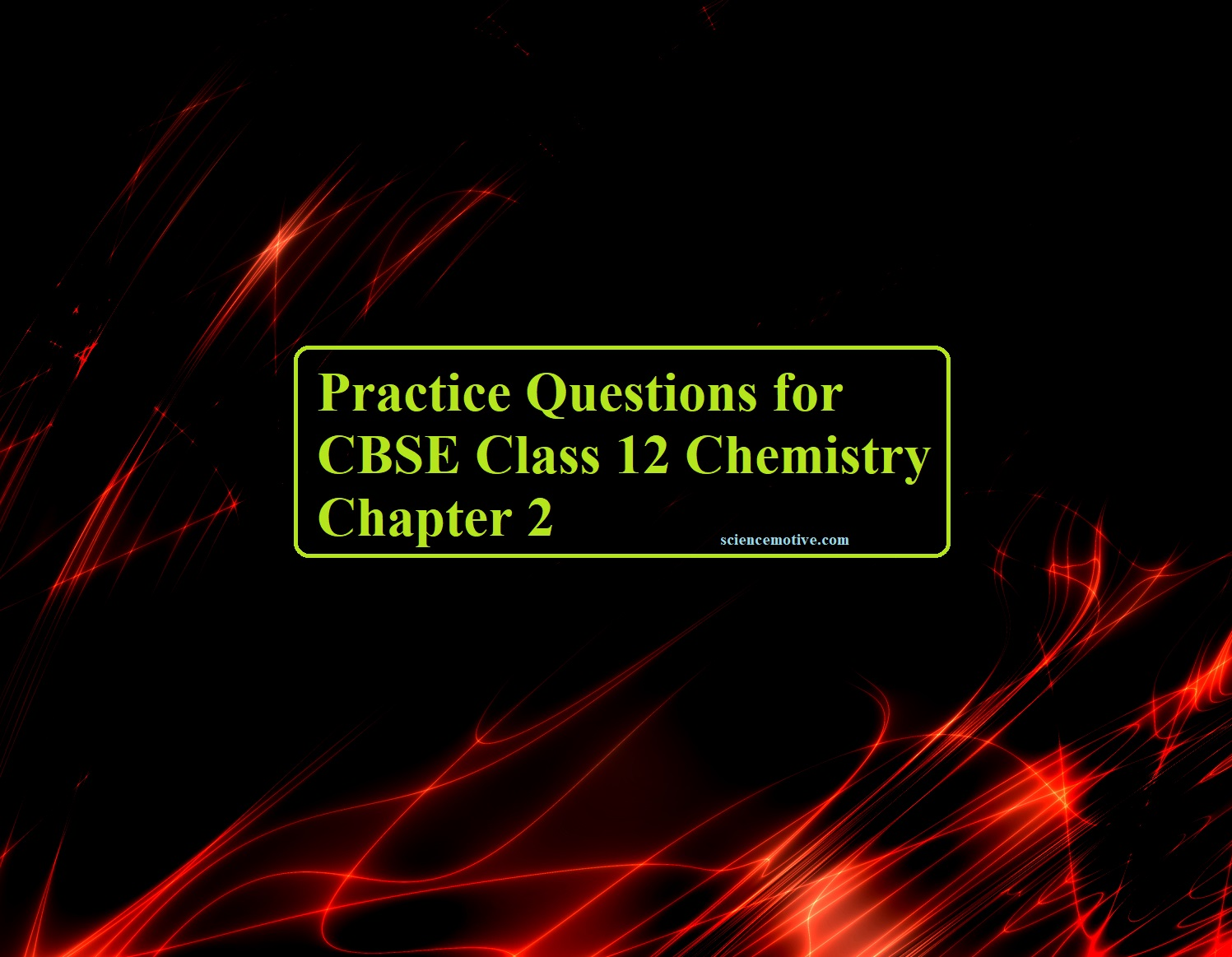# Practice Questions for CBSE Class 12 Chemistry Chapter 2# Practice Questions for CBSE Class 12 Chemistry Chapter 2

Class 12 Chemistry Chapter 2

1. Which type of solutes shows colligative properties, volatile or non-volatile?

2. What is the sign of (ΔG)sol for a non-ideal solution?

3. What is the sign of (ΔS) for ideal solutions?

4. Why do gases nearly always tend to be less soluble in liquid as the temperature is raised? partnersuche ab 60 in zug

5. Calculate the mole fractions in the solution made by dissolving 25 g of potassium hydroxide (KOH) in 200 mL of water.

6. When acetone is mixed with chloroform, a reduction in volume takes place. What type of deviation from Raoult’s law is shown in this case?

7. The dissolution of NH4Cl in water is an endothermic process that will be the effect of temperature on its solubility.

8. Why is the vapour pressure of a solution of glucose in water lower than that of water?

9. Henry’s law constant for the solubility of N2 in water at 25°C is 105 atm and the air is supposed to be a 1:4 mixture of O2 and N2. Determine the number of moles of air dissolved in 1-mole water at 5 atm pressure.

10. After removing the shell of the egg, one is placed in distilled water while the other is placed in a saturated solution of NaCl. obwalden sexkontakte What will you observe and why?

11. What type of liquids from ideal solutions?

12. It is advised to add ethylene glycol to water in car radiation while driving in the hill station. Why?

13. What will be the value of van’t Hoff’s factor for a dilute solution of K2SO4 in water?

14. Calculate the osmotic pressure of 0.1 M dibasic strong acid of pH = 4 at 27°C.

15. 1-mole urea and 1 mole NaCl are added to water. Kf = 1.86, Kb = 0.52 (ln K kg mole–1) determine the ratio of ΔTb vs ΔTf for the solution.

16. Define osmosis and the conditions for isotonic solutions.

17. Vapour pressure of dilute aq. solution of urea is 740 mm Hg at 373 K. Calculate (i) Molality and (ii) xsolute.

18. Calculate the mass of urea required in making 2.5 kg of 0.25 molal aqueous solution.

19. Calculate the more fraction of ethylene glycol (C2H6O2) in a solution containing 30% of C2H6O2 by mass.

20. Two liquids A and B form an ideal solution at 323 K. frauenfeld partnervermittlung agentur A liquid mixture containing one mole of A and two moles of b has a vapour pressure of 250 m bar. If one more mole of A is added to the solution, the vapour pressure increases to 300 m bar. Calculate the vapour pressures of liquids A and B at 323 K.

Class 12 Chemistry Chapter 2